Win up to 100% scholarship on Aakash BYJU'S JEE/NEET courses with ABNAT Win up to 100% scholarship on Aakash BYJU'S JEE/NEET courses with ABNAT

# JEE Main 2022 June 26 – Shift 1 Physics Question Paper with Solutions

Students can find the JEE Main 2022 June 26 – Shift 1 Physics Question Paper with Solutions here. The questions are based on the Physics curriculum for Classes XI and XII. Candidates may access the PDF format of the question papers on this page. The PDF download can also be used by the students for future reference. The JEE experts at BYJU’S have authored these solutions. Candidates can improve their JEE marks by studying the JEE Main 2022 Question Papers. Additionally, by using this JEE Main 2022 answer key as a guide, applicants can effectively get ready for the upcoming exam.

## JEE Main 2022 Physics Question Paper with Solutions June 26 – Shift 1

JEE Main 2022 June 26 – Shift 1 Physics Question Paper with Solutions

SECTION – A

Multiple Choice Questions: This section contains 20 multiple choice questions. Each question has 4 choices (1), (2), (3) and (4), out of which ONLY ONE is correct.

1. An expression for a dimensionless quantity P is given by

$$\begin{array}{l}P=\frac{\alpha}{\beta}log_e\left ( \frac{kt}{\beta x} \right )\end{array}$$
; where α and β are constants, x is distance; k is Boltzmann constant and t is the temperature. Then the dimensions of α will be

(A) [M0L–1T0]

(B) [ML0T–2]

(C) [MLT–2]

(D) [ML2T–2]

Sol.

$$\begin{array}{l}\left [ \alpha \right ]=\left [ \beta \right ]=\left [ \frac{kt}{x} \right ]\end{array}$$
$$\begin{array}{l}=\left [ \frac{ML^2T^{-2}}{L} \right ]\end{array}$$

= [MLT–2]

2. A person is standing in an elevator. In which situation, he experiences weight loss?

(A) When the elevator moves upward with constant acceleration

(B) When the elevator moves downward with constant acceleration

(C) When the elevator moves upward with uniform velocity

(D) When the elevator moves downward with uniform velocity

Sol. Apparent weight = m(ga)

⇒ Weight loss in downward accelerated elevator

3. An object is thrown vertically upwards. At its maximum height, which of the followingquantity becomes zero?

(A) Momentum

(B) Potential Energy

(C) Acceleration

(D) Force

Sol. At topmost position,

v = 0

⇒ momentum = 0

4. A ball is released from rest from point P of a smooth semi-spherical vessel as shown in figure.The ratio of the centripetal force and normal reaction on the ball at point Q is A whileangular position of point Q is α with respect to point P. Which of the following graphsrepresent the correct relation between A and α when ball goes from Q to R?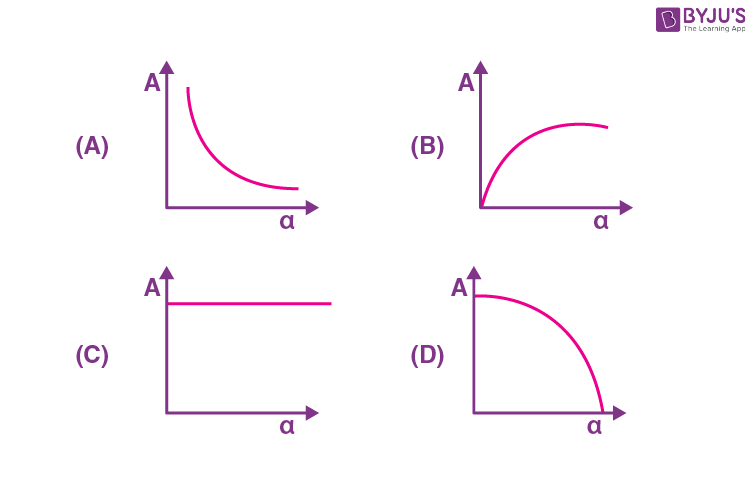Sol.

$$\begin{array}{l}N=mg\sin \alpha+\frac{mv^2}{R}\end{array}$$

and, v2 = 2g × Rsinα

N = mgsinα + m × (2gsinα)

= 3mgsinα

$$\begin{array}{l}\textup{ratio},~A=\frac{\frac{mv^2}{R}}{N}\end{array}$$
$$\begin{array}{l}=\frac{2mg~\sin\alpha}{3mg~\sin\alpha}\end{array}$$
$$\begin{array}{l}=\frac{2}{3}\end{array}$$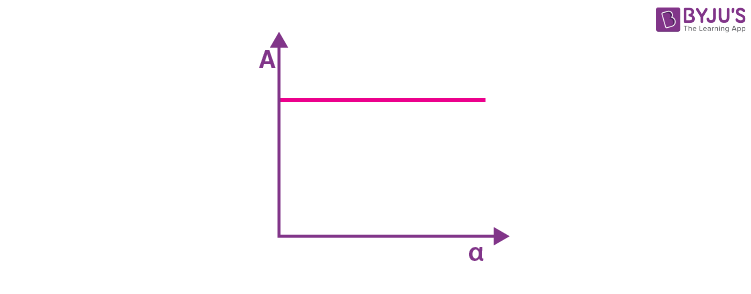5. A thin circular ring of mass M and radius R is rotating with a constant angular velocity 2 rad s–1 in a horizontal plane about an axis vertical to its plane and passing through the center of the ring. If two objects each of mass m be attached gently to the opposite ends of a diameter of ring, the ring will then rotate with an angular velocity (in rad s–1).

(A)

$$\begin{array}{l}\frac{M}{\left ( M+m \right )}\end{array}$$

(B)

$$\begin{array}{l}\frac{\left ( M+2m \right )}{2M}\end{array}$$

(C)

$$\begin{array}{l}\frac{2M}{\left ( M+2m \right )}\end{array}$$

(D)

$$\begin{array}{l}\frac{2\left ( M+2m \right )}{M}\end{array}$$

Sol.

l1ω1 = l2ω2

$$\begin{array}{l}MR^2\times2=\left ( MR^2+2mR^2 \right )\omega_2\end{array}$$

$$\begin{array}{l}\omega_2=\frac{2M}{M+2m}\end{array}$$

6. The variation of acceleration due to gravity (g) with distance (r) from the center of the earth is correctly represented by

(Given R = radius of earth)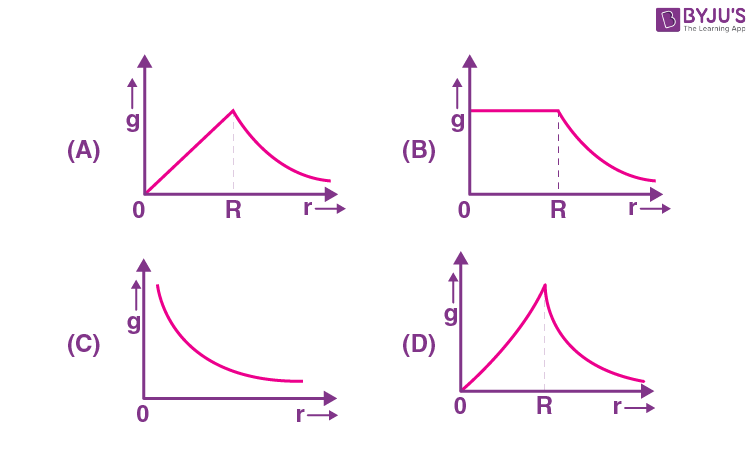Sol. For r < R

$$\begin{array}{l}g=\frac{Gmr}{R^3}=Cr~\left ( C=\textup{Constant} \right )\end{array}$$

For r > R

$$\begin{array}{l}g=\frac{Gm}{r^2}=\frac{C^{‘}}{r^2}\left ( C^{‘}=\textup{Constant} \right )\end{array}$$

For the above equations the best suited graph is as given in option (A)

7. The efficiency of a Carnot’s engine, working between steam point and ice point, will be

(A) 26.81%

(B) 37.81%

(C) 47.81%

(D) 57.81%

Sol.

$$\begin{array}{l}\eta=1-\frac{T_C}{T_H} =\frac{T_H-T_C}{T_H}\end{array}$$
$$\begin{array}{l}=\frac{100}{373}\times100 %\end{array}$$

= 26.81%

⇒ option (A)

8. Time period of a simple pendulum in a stationary lift is ‘T’. If the lift accelerates with

$$\begin{array}{l}\frac{g}{6}\end{array}$$
vertically upwards then the time period will be

(Where g = acceleration due to gravity)

(A)

$$\begin{array}{l}\sqrt{\frac{6}{5}}T \end{array}$$

(B)

$$\begin{array}{l}\sqrt{\frac{5}{6}}T \end{array}$$

(C)

$$\begin{array}{l}\sqrt{\frac{6}{7}}T \end{array}$$

(D)

$$\begin{array}{l}\sqrt{\frac{7}{6}}T \end{array}$$

Sol.

$$\begin{array}{l}T^{‘}=2\pi\sqrt{\frac{I}{g_{eff}}} \end{array}$$
$$\begin{array}{l}T^{‘}=2\pi\sqrt{\frac{I}{g+\frac{g}{6}}}=2\pi\sqrt{\frac{6I}{7g}}\end{array}$$

$$\begin{array}{l}T^{‘}=\sqrt{\frac{6}{7}}T\end{array}$$

9. A thermally insulated vessel contains an ideal gas of molecular mass M and ratio of specific heats 1.4. Vessel is moving with speed ν and is suddenly brought to rest. Assuming no heat is lost to the surrounding and vessel temperature of the gas increases by

(R = universal gas constant)

(A)

$$\begin{array}{l}\frac{Mv^2}{7R}\end{array}$$

(B)

$$\begin{array}{l}\frac{Mv^2}{5R}\end{array}$$

(C)

$$\begin{array}{l}2\frac{Mv^2}{7R}\end{array}$$

(D)

$$\begin{array}{l}7\frac{Mv^2}{5R}\end{array}$$

Sol.

$$\begin{array}{l}\frac{1}{2}mv^2=n\frac{5}{2}R\Delta T\end{array}$$

$$\begin{array}{l}\Delta T=\frac{mv^2}{5nR}\end{array}$$
$$\begin{array}{l}=\frac{MV^2}{5R}\end{array}$$

Option (B)

10. Two capacitors having capacitance C1 and C2 respectively are connected as shown in figure. Initially, capacitor C1 is charged to a potential difference V volt by a battery. The battery is then removed and the charged capacitor C1 is now connected to uncharged capacitor C2 by closing the switch S. The amount of charge on the capacitor C2, after equilibrium, is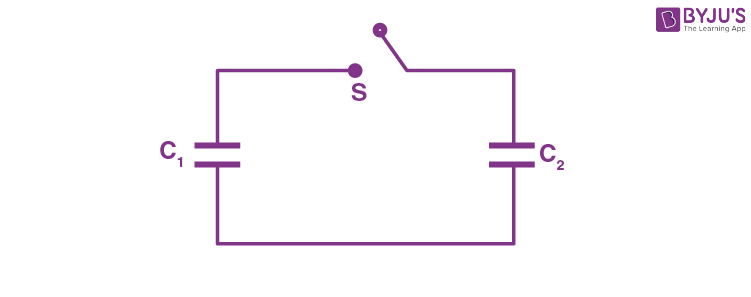(A)

$$\begin{array}{l}\frac{C_1C_2}{\left ( C_1+C_2 \right )}V\end{array}$$

(B)

$$\begin{array}{l}\frac{\left ( C_1+C_2 \right ) }{C_1C_2}V\end{array}$$

(C) (C1 + C2)V

(D) (C1C2)V

Sol.

$$\begin{array}{l}V_{\textup{common}}=\frac{C_1V}{C_1 + C_2}\end{array}$$

⇒ Charge on capacitor C2

= C2Vcommon

$$\begin{array}{l}=\frac{C_1C_2V}{C_1+C_2}\end{array}$$

⇒ Option (A)

11. Given below two statements: One is labelled as Assertion (A) and other is labelled as Reason (R).

Assertion (A) : Non-polar materials do not have any permanent dipole moment.

Reason (R) :When a non-polar material is placed in an electric field, the centre of the positive charge distribution of it’s individual atom or molecule coincides with the centre of the negative charge distribution.

In the light of above statements, choose the most appropriate answer from the options given below.

(A) Both (A) and (R) are correct and (R) is the correct explanation of (A).

(B) Both (A) and (R) are correct and (R) is not the correct explanation of (A).

(C) (A) is correct but (R) is not correct.

(D) (A) is not correct but (R) is correct.

Sol. Non polar material does not have any permanent dipole moment and when placed in an electric field the positive and negative charges displace in opposite directions and result into an induced dipole moment as long as the field is applied.

12. The magnetic flux through a coil perpendicular to its plane is varying according to the relation
φ = (5t3 + 4t2 + 2t – 5) Weber. If the resistance of the coil is 5 ohm, then the induced current through the coil at t = 2 s will be,

(A) 15.6 A

(B) 16.6 A

(C) 17.6 A

(D) 18.6 A

Sol.

$$\begin{array}{l}\text{Emf}=-\frac{d\phi}{dt}=-\left ( 15t^2+8t+2 \right ) \end{array}$$

So,

$$\begin{array}{l}i=\frac{\left| \textup{Emf}\right|}{R}=\frac{\left ( 15t^2+8t+2 \right )}{5}\end{array}$$

at t = 2

i = 15.6 A

13. An aluminium wire is stretched to make its length, 0.4% larger. The percentage change in resistance is :

(A) 0.4%

(B) 0.2%

(C) 0.8%

(D) 0.6%

Sol. When the wire is stretched, volume remains constant. If length is increased by 0.4% area will decrease by 0.4% so

From

$$\begin{array}{l}R=\rho\frac{I}{A}\end{array}$$
$$\begin{array}{l}\frac{dR}{R}\times100=\frac{dI}{I}\times100+\frac{dA}{A}\times 100\end{array}$$

%R = 0.4 + 0.4 = 0.8%

14. A proton and an alpha particle of the same velocity enter in a uniform magnetic field which is acting perpendicular to their direction of motion. The ratio of the radii of the circular paths described by the alpha particle and proton is :

(A) 1:4

(B) 4:1

(C) 2:1

(D) 1:2

Sol.

$$\begin{array}{l}R=\frac{mv}{qB}\end{array}$$
$$\begin{array}{l}\frac{R_\alpha}{R_p}=\frac{m_\alpha/q_\alpha}{m_p/q_q}=2\end{array}$$

15. If electric field intensity of a uniform plane electro magnetic wave is given as

$$\begin{array}{l}E=-301.6\sin\left ( kz-\omega t \right )\hat{a}_x+452.4\sin \left ( kz-\omega t \right )\hat{a}_y\frac{V}{m} \end{array}$$

Then, magnetic intensity ‘H’ of this wave in Am–1 will be :

[Given : Speed of light in vacuum c = 3 × 108ms–1, Permeability of vacuum μ0 = 4π × 10–7 NA–2]

(A)

$$\begin{array}{l}+0.8 \sin\left ( kz-\omega t \right )\hat{a}_y+0.8\sin\left ( kz-\omega t \right )\hat{a}_x\end{array}$$

(B)

$$\begin{array}{l}+1.0\times10^{-6}\sin\left ( kz-\omega t \right )\hat{a}_y+1.5\times10^{-6} \left ( kz-\omega t \right )\hat{a}_x\end{array}$$

(C)

$$\begin{array}{l}-0.8\sin\left ( kz-\omega t \right )\hat{a}_y-1.2\sin\left ( kz-\omega t \right )\hat{a}_x\end{array}$$

(D)

$$\begin{array}{l}-1.0\times10^{-6}\sin\left ( kz-\omega t \right )\hat{a}_y-1.5\times10^{-6} \sin\left ( kz-\omega t \right )\hat{a}_x\end{array}$$

Sol. We know

$$\begin{array}{l}\vec{B}\times\vec{C}=\vec{E}\end{array}$$

Taking cross product of vector C both the sides

$$\begin{array}{l}\vec{C}\times\left ( \vec{B}\times\vec{C} \right )=\vec{C}\times\vec{E}\end{array}$$

So

$$\begin{array}{l}\vec{B}=\frac{\vec{C}\times\vec{E}}{C^2}\end{array}$$
$$\begin{array}{l}\vec{C}=C\hat{k}\end{array}$$
$$\begin{array}{l}\vec{E}=-301.6\sin\left ( kz-\omega t \right )\hat{a}_x+452.4\sin\left ( kz-\omega t \right )\hat{a}_y\end{array}$$

and

$$\begin{array}{l}\vec{H}=\frac{\vec{B}}{\mu_0}\end{array}$$

On solving

$$\begin{array}{l}\vec{H}=-0.8\sin\left ( kz-\omega t \right )\vec{a}_y-1.2\sin\left ( kz-\omega t \right )\vec{a}_x\end{array}$$

16. In free space, an electromagnetic wave of 3 GHz frequency strikes over the edge of an object of size

$$\begin{array}{l}\frac{\lambda}{100}\end{array}$$
, whereλ is the wavelength of the wave in free space. The phenomenon, which happens there will be:

(A) Reflection

(B) Refraction

(C) Diffraction

(D) Scattering

Sol. Since size is of the order of

$$\begin{array}{l}\frac{\lambda}{100}\end{array}$$
, hence scattering will take place.

17. An electron with speed υ and a photon with speed c have the same de-Broglie wavelength. If the kinetic energy and momentum of electron are Ee and pe and that of photon are Eph and pph respectively. Which of the following is correct?

(A)

$$\begin{array}{l}\frac{E_e}{E_{ph}}=\frac{2c}{\nu }\end{array}$$

(B)

$$\begin{array}{l}\frac{E_e}{E_{ph}}=\frac{\nu }{2c}\end{array}$$

(C)

$$\begin{array}{l}\frac{p_e}{p_{ph}}=\frac{2c}{\nu}\end{array}$$

(D)

$$\begin{array}{l}\frac{p_e}{p_{ph}}=\frac{\nu}{2c}\end{array}$$

Sol.

$$\begin{array}{l}\lambda_e=\lambda_{ph}\Rightarrow\frac{h}{p_e}=\frac{hc}{E_{ph}}\end{array}$$
$$\begin{array}{l}\Rightarrow E_{ph}=p_e\times c =2E_e\frac{c}{\nu}\end{array}$$

$$\begin{array}{l}\frac{E_e}{E_{ph}}=\frac{\nu}{2c}\end{array}$$

18. How many alpha and beta particles are emitted when Uranium 92U238 decays to lead 82Pb206?

(A) 3 alpha particles and 5 beta particles

(B) 6 alpha particles and 4 beta particles

(C) 4 alpha particles and 5 beta particles

(D) 8 alpha particles and 6 beta particles

Sol.

$$\begin{array}{l}_{92}^{238}\cup\xrightarrow{~~~~~}_{82}^{206}Pb+x_2^4He+y\left ( _{-1}^0e \right )\end{array}$$

⇒ 206 + 4x = 238 …(1)

and 82 + 2xy = 92 …(2)

x = 8 and y = 6

19. The IV characteristics of a p-n junction diode in forward bias is shown in the figure. The ratio of dynamic resistance, corresponding to forward bias voltage of 2 V and 4 V respectively, is :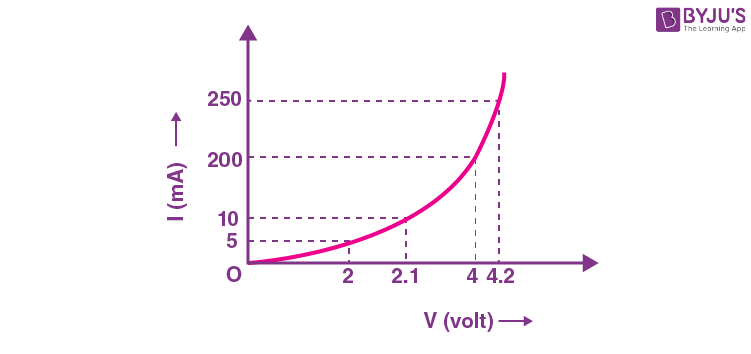(A) 1 : 2

(B) 5 : 1

(C) 1 : 40

(D) 20 : 1

Sol. Dynamic resistance

$$\begin{array}{l}=\frac{dV}{dl}\end{array}$$

$$\begin{array}{l}r_1=\frac{2.1-2}{10-5}k\Omega\end{array}$$

&

$$\begin{array}{l}r_2=\frac{4.2-4}{250-200}k\Omega\end{array}$$

r1 :r2 = 5 : 1.

20. Choose the correct statement for amplitude modulation :

(A) Amplitude of modulating signal is varied in accordance with the information signal.

(B) Amplitude of modulated signal is varied in accordance with the information signal.

(C) Amplitude of carrier signal is varied in accordance with the information signal.

(D) Amplitude of modulated signal is varied in accordance with the modulating signal.

Sol. In amplitude modulation, amplitude of carrier signal is varied according to the message signal.

SECTION – B

Numerical Value Type Questions: This section contains 10 questions. In Section B, attempt any five questions out of 10. The answer to each question is a NUMERICAL VALUE. For each question, enter the correct numerical value (in decimal notation, truncated/rounded-off to the second decimal place; e.g. 06.25, 07.00, –00.33, –00.30, 30.27, –27.30) using the mouse and the on-screen virtual numeric keypad in the place designated to enter the answer.

1. A fighter jet is flying horizontally at a certain altitude with a speed of 200 ms–1. When it passes directly overhead an anti-aircraft gun, a bullet is fired from the gun, at an angle θ with the horizontal, to hit the jet. If the bullet speed is 400 m/s, the value of θ will be ____°.

Sol.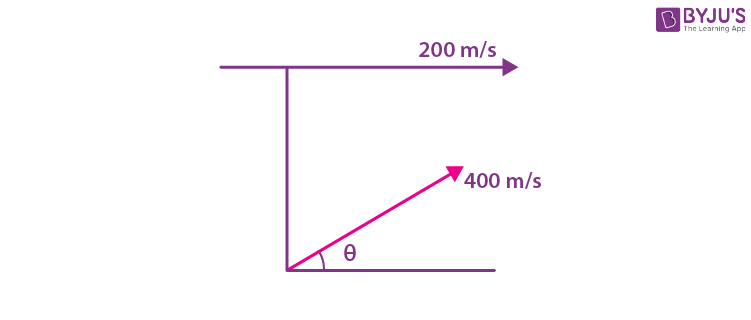To hit the jet

400 cosθ = 200

$$\begin{array}{l}\cos\theta=\frac{1}{2}\end{array}$$

⇒ θ = 60°

2. A ball of mass 0.5 kg is dropped from the height of 10 m. The height, at which the magnitude of velocity becomes equal to the magnitude of acceleration due to gravity, is ___ m.

[Use g = 10 m/s2]

Sol. gt = g

t = 1 sec

$$\begin{array}{l}\Delta h=\frac{1}{2}gt^2=\frac{1}{2}\times5\times1^2=5~\text{m}\end{array}$$

h = H – Δh

= 10 – 5

= 5 m

3. The elastic behaviour of material for linear stress and linear strain, is shown in the figure. The energy density for a linear strain of 5 × 10–4 is ____ kJ/m3. Assume that material is elastic upto the linear strain of 5 × 10–4.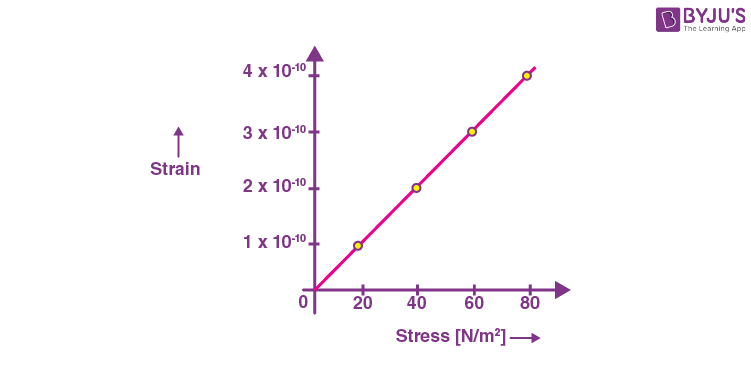Sol.

$$\begin{array}{l}u_d=\frac{1}{2}\times Y\times\left ( \textup{strain} \right )^2\end{array}$$
$$\begin{array}{l}=\frac{1}{2}\times\left ( \frac{20}{10^{-10}} \right )\times\left ( 5\times10^{-4} \right )^2\end{array}$$

= 1011 × 25 × 10–8

= 25 × 103 J/m3

= 25 kJ/m3

4. The elongation of a wire on the surface of the earth is 10–4m. The same wire of same dimensions is elongated by 6 × 10–5m on another planet. The acceleration due to gravity on the planet will be ___ ms–2. (Take acceleration due to gravity on the surface of earth = 10 ms–2)

Sol.

$$\begin{array}{l}\Delta I=\frac{M^{‘}gI}{2A~y}\end{array}$$

⇒ Δl g

$$\begin{array}{l}\frac{g_p}{g_e}=\frac{\Delta I_p}{\Delta I_e}=\frac{6\times 10^{-5}}{10\times10^{-5}} \end{array}$$

gp = 6 m/s2 as ge = 10 m/s2

5. A 10 Ω, 20 mH coil carrying constant current is connected to a battery of 20 V through a switch. Now after switch is opened current becomes zero in 100 μs. The average e.m.f. induced in the coil is ____V.

Sol. Initial flux through inductor = LI

$$\begin{array}{l}\phi_i=20\times 10^{-3}\times\frac{20}{10}\end{array}$$

= 4 × 10–2 weber

Final flux = 0

⇒ average emf

$$\begin{array}{l}=\frac{\left|\phi_i-\phi_f \right|}{100~\mu \textup{s}}\end{array}$$
$$\begin{array}{l}=\frac{4\times10^{-2}}{10^{-4}}=400~\text{V}\end{array}$$

6. A light ray is incident, at an incident angle θ1, on the system of two plane mirrors M1 and M2 having an inclination angle 75° between them (as shown in figure). After reflecting from mirror M1 it gets reflected back by the mirror M2 with an angle of reflection 30°. The total deviation of the ray will be ________ degree.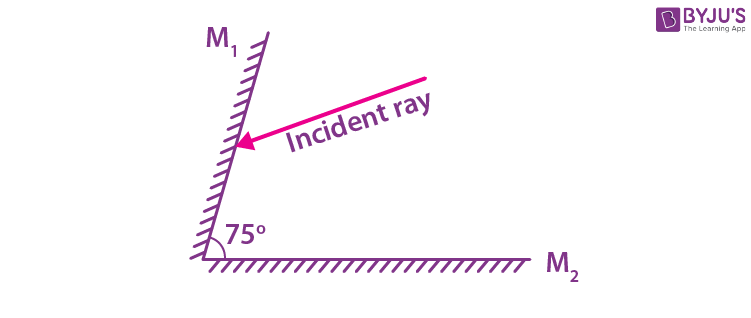Sol.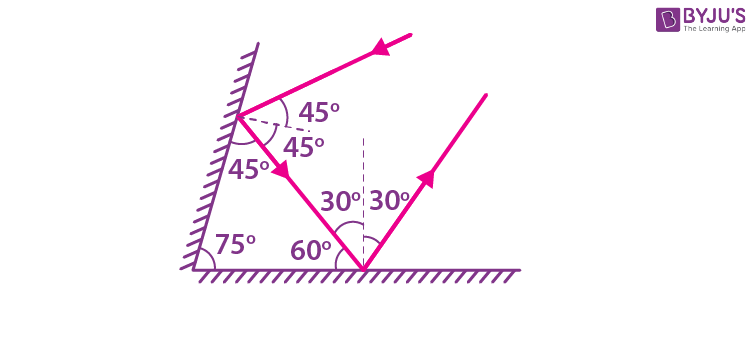On first reflection angel of deviation is 90° and on second reflection angle of deviation is 120°

so total deviation is δ = 90° +120° = 210°

7. In a vernier callipers, each cm on the main scale is divided into 20 equal parts. If tenth vernier scale division coincides with nineth main scale division. Then the value of vernier constant will be
____ ×10–2 mm.

Sol.

$$\begin{array}{l}LC=\frac{1MSD}{VSD}=\frac{\frac{1}{20}\textup{cm}}{10}\end{array}$$
$$\begin{array}{l}=\frac{1}{200}\textup{cm}\end{array}$$

= 5 × 10–2 mm

8. As per the given circuit, the value of current through the battery will be ______ A.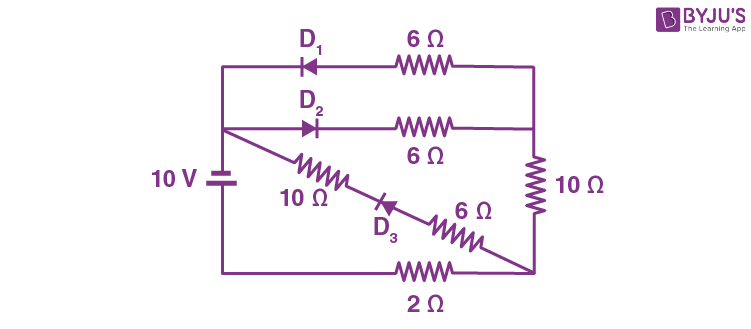Sol. Because of diode D2 current will not flow through it so new circuit diagram is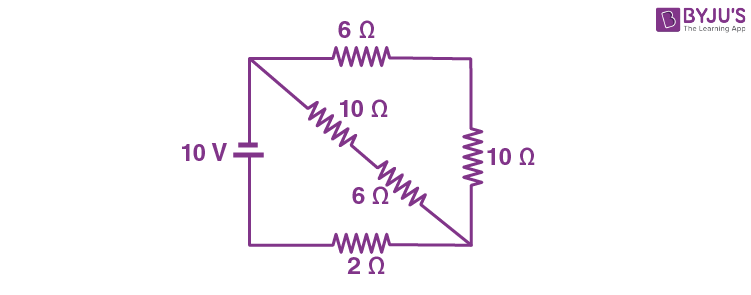so Rnet =10 Ω

and

$$\begin{array}{l}i=\frac{V}{R_{net}}=1A\end{array}$$

9. A 110 V,50 Hz,AC source is connected in the circuit (as shown in figure). The current through the resistance 55Ω, at resonance in the circuit, will be _______ A.Sol. At resonance

$$\begin{array}{l}\left ( \omega=\frac{1}{\sqrt{LC}} \right )\end{array}$$
, impedance of the circuit is infinite.

⇒Current through resistance = 0.

10. An ideal fluid of density 800kgm–3, flows smoothly through a bent pipe (as shown in figure) that tapers in cross-sectional area from a to

$$\begin{array}{l}\frac{a}{2}\end{array}$$
. The pressure difference between the wide and narrow sections of pipe is 4100 Pa. At wider section, the velocity of fluid is
$$\begin{array}{l}\frac{\sqrt{x}}{6}\end{array}$$
ms–1 for x=_______. (Given g=10 ms–2)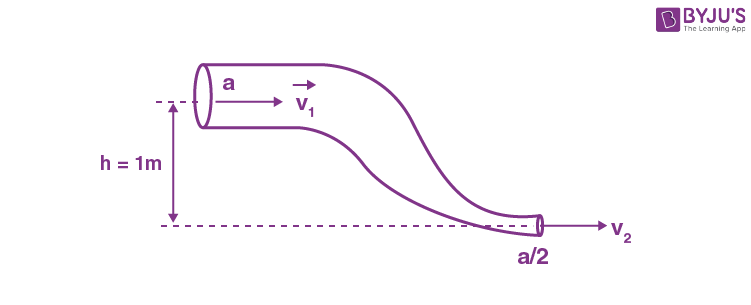$$\begin{array}{l}P_1+\rho gh+\frac{1}{2}\rho v^2 = P_2+0 +\frac{1}{2}\rho\left ( 2v \right )^2\end{array}$$
$$\begin{array}{l}4100=800\left\{ \frac{3}{2}v^2-10\right\}\end{array}$$
$$\begin{array}{l}\Rightarrow v=\frac{\sqrt{363}}{6}\ m/s\end{array}$$